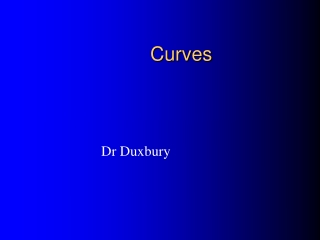DownloadDownload PresentationCurves

# Curves

Télécharger la présentation## Curves

- - - - - - - - - - - - - - - - - - - - - - - - - - - E N D - - - - - - - - - - - - - - - - - - - - - - - - - - -
##### Presentation Transcript

1. Curves Dr Duxbury

2. Quadratic graphs In a quadratic function, the highest power of x is 2 and it is of the form y = ax2 + bx + c. Are the following quadratic functions?

3. Cubic graphs In a cubic function, the highest power of x is 3 and it is of the form y = ax3 + bx2 + cx + d. Are the following cubic functions?

4. Drawing a quadratic graph Plot the following quadratic function: What do we do first?

5. Set up a table finding points which are on the curve:

6. Set up a table finding points which are on the curve:

7. Set up a table finding points which are on the curve:

8. Set up a table finding points which are on the curve:

9. Set up a table finding points which are on the curve:

11. Graphical Solution of Equations Solve Let Then find the values of x where the curve cuts the x-axis (that is where y=0).

12. Graphical Solution of Equations If we were to solve this equation without using a graph, then we could factorise the equation: To solve it graphically:

13. y The roots of a quadratic equation Solve x2 + 2x – 3 = 0 x = -3 x = 1

14. The roots of an equation This tells us that the solution to the equation is

15. y The roots of a quadratic equation Solve x2 + 2x – 3 = -2 So x = -2.5 and 0.5 x = 0.5 x = -2.5 y = -2

16. Exercise: Plot the graph of y = x2 +3x – 4 for values of x between –5 and 2 Use your graph to find values of x such that x2 +3x – 4 = 0, x2 +3x – 4 = -4 and x2 +3x – 4 = x

17. Solution y = 0

18. Solution y = -4

19. Solution y = x